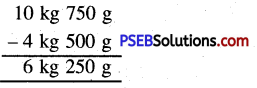# PSEB 5th Class Maths Solutions Chapter 6 Measurement Ex 6.4

Punjab State Board PSEB 5th Class Maths Book Solutions Chapter 6 Measurement Ex 6.4 Textbook Exercise Questions and Answers.

## PSEB Solutions for Class 5 Maths Chapter 6 Measurement Ex 6.4

Question 1.
7 km 7.50 m and 2 km 575 m
Solution: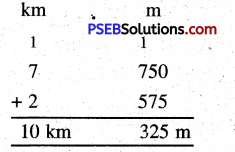= 10 km 325 m

Question 2.
4 kg 500 g and 9 kg 825 g
Solution: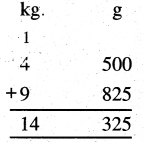= 14 kg 325 kgQuestion 3.
5 l 925 ml and 7 l 650 ml
Solution: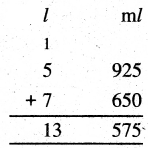= 13 l 575 ml

Question 4.
10 m, 3 m 85 cm and 6 m 25 cm
Solution: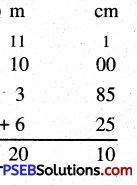= 20 m 10 cm

Question 5.
8 kg 700 g, 975 g and 2 kg 350 g
Solution: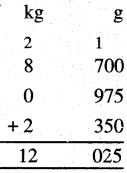= 12 kg 25 g

2. Subtract:

Question 1.
7 km 625 m from 12 km 300 m
Solution: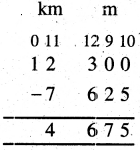= 4 km 675 mQuestion 2.
3 kg 650 g from 8 kg
Solution: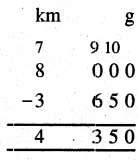= 4 kg 350 g

Question 3.
5 l 850 ml from 10 l 350 ml
Solution: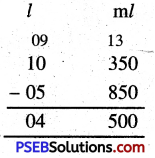= 4 l 500 ml

Question 4.
9m 60 cm from 15 m
Solution: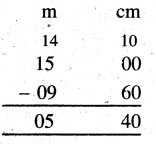= 5 m 40 cmQuestion 5.
13 l from 25 l 765 ml
Solution: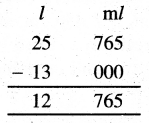= 12 l 765 ml

Question 3.
Anand has bought 2 kg 350 g onions. 1 kg 750 g potatoes. How many kilograms of vegetables has he bought?
Solution:
Quantity of onions bought by Anand = 2 kg 350 g
Quartity of potatoes bought by Anand = 1 kg 750 g
Total quantity of vegetables bought = 4 kg 100 g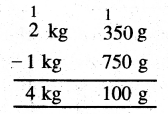Question 4.
Ajay has travelled 150 km 400 m distancebybus, 120 km 650 m by taxi. How much distance has he covered?
Solution:
Distance travelled by bus = 150 km 400 m
Distance travelled by taxi = 120 km 650 m
Total distance travelled by him = 271 km 50 m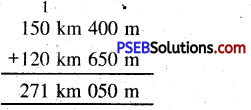Question 5.
Three containers contained 10 l 350 ml, 9 l 850 ml and 11 l oil respectively. Find the total quantity of oil contained in three containers.
Solution:
First container contains oil = 10 l 350 ml
Second container contains oil = 9 l 850 ml
Third container contains oil = 11 l 000 ml
The total quantity of oil contained in three containers = 31 l 200 ml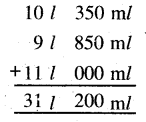Question 6.
Anita bought 7 m 30 cm cloth. She used 2 m 50 cm cloth for her suit. Find the remaining length of the cloth.
Solution:
Length of cloth bought by Anita = 7 m 30 cm
Length of cloth used for suit = 2 m 50 cm
Remaining length of cloth = 4 m 80 cm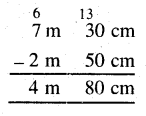Question 7.
A family consumes 10 kg 750 g wheat and 4 kg 500 g rice in a month. Find the difference of consumption of rice and wheat.
Solution:
Quantity of wheat consumed = 10 kg 750 g
Quantity of rice consumed = 4 kg 500 g
Difference of consumption of rice and wheat = 6 kg 250 g